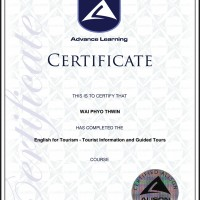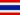# 4.2 Introducción de datos

• Apuntes
• Revisión por temaWAI P. 0 0 Formulas and functions. Formulas and functions are mathematical equations. The numeric value of formulas and functions automatically changes when the numeric value of variables associated with them changes.WAI P. 0 0 How many types of formula?